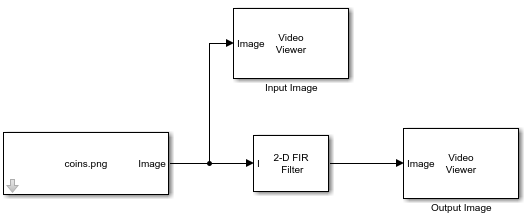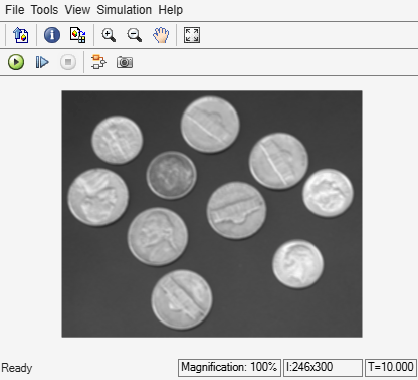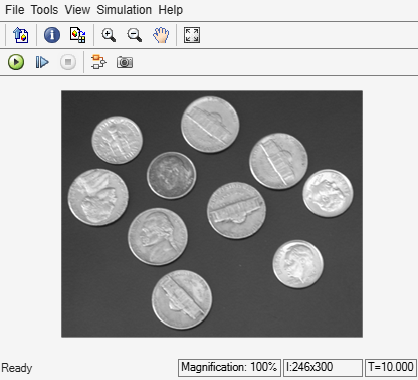# Filter Image Using FIR Filter

This example shows you how to filter an image using an FIR filter.

### Create the FIR Filter

Create a 3-by-3 average filter to smooth an image.

`H = fspecial("average",3)`
```H = 3×3 0.1111 0.1111 0.1111 0.1111 0.1111 0.1111 0.1111 0.1111 0.1111 ```

Using separable filter coefficients reduces the number of calculations required to compute the output. Check if the filter `H` is separable, and compute the vertical and horizontal filter coefficients.

`[sep,HV,HH] = isfilterseparable(H)`
```sep = logical 1 ```
```HV = 3×1 -0.3333 -0.3333 -0.3333 ```
```HH = 1×3 -0.3333 -0.3333 -0.3333 ```

### Example Model

```modelname = "ex_blk2DFIRFilter.slx"; open_system(modelname)```The model reads a PNG image using an Image From File block with the File name parameter set to `coins.png`. To filter the input image, the model uses a 2-D FIR Filter block with the Separable filter coefficients option selected, Vertical coefficients (across height) parameter set to `HV`, Horizontal coefficients (across width) parameter set to `HH`, and the Output size parameter set to `Same as input port I`.

### Simulate the Model

Run the model. The model displays the input image and the filtered output image using Video Viewer blocks.

`output = sim(modelname)````output = Simulink.SimulationOutput: tout: [51x1 double] SimulationMetadata: [1x1 Simulink.SimulationMetadata] ErrorMessage: [0x0 char] ```